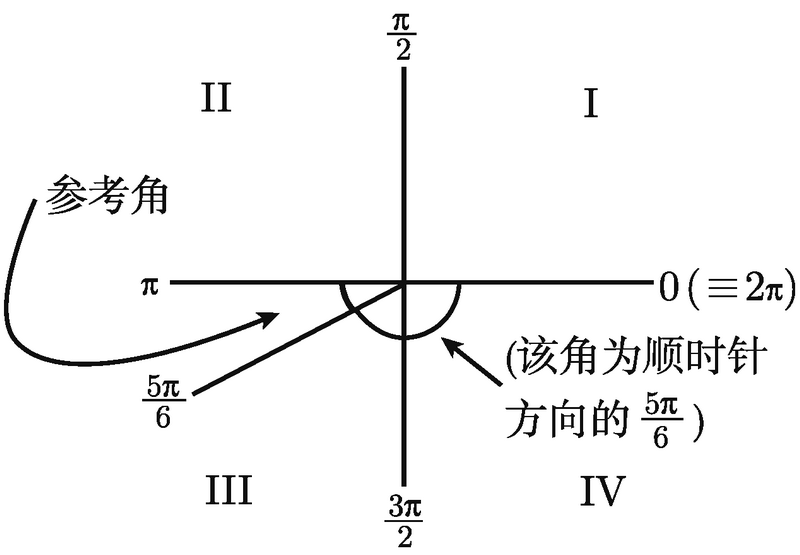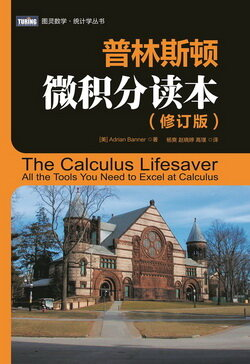# 普林斯顿微积分读本（修订版）(18)：三角学回顾 2.2.2([0, 2π] 以外的三角函数)

$\frac{\pi}{2},\frac{5\pi}{2},\frac{9\pi}{2},\frac{13\pi}{2},\frac{17\pi}{2},\cdots.$

$\cdots,-\frac{15\pi}{2},-\frac{11\pi}{2},-\frac{7\pi}{2},-\frac{3\pi}{2},\frac{\pi}{2},\frac{5\pi}{2},\frac{9\pi}{2},\frac{13\pi}{2},\frac{17\pi}{2},\cdots.$